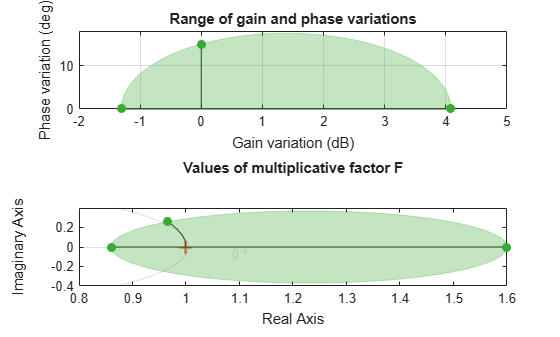# plot

Visualize gain and phase uncertainty of a `umargin` block

## Syntax

``plot(ublk)``

## Description

example

````plot(ublk)` generates a two-axis plot visualizing the gain and phase uncertainty modeled by a `umargin` block. The plot shows:The ranges of modeled gain and phase uncertainty. The plot shows the maximum gain-only variation, the maximum phase-only variation, and a shaded region containing the simultaneous gain and phase variations represented by `ublk`.The disk of values taken by the dynamic, multiplicative factor F(s) that models the gain and phase uncertainty. For SISO loops, this factor changes the open-loop response L to L*F. For more information about the disk model of uncertainty, see Stability Analysis Using Disk Margins.```

## Examples

collapse all

Create a `umargin` block that models gain that can decrease by 10% but increase by 60% in the absence of phase variation, and a phase variation of ±15° in the absence of gain variation.

```DGM = getDGM([0.9,1.6],[-15,15],'tight'); F = umargin('F',DGM)```
```F = Uncertain gain/phase "F" with relative gain change in [0.86,1.6] and phase change of ±15 degrees. ```

Visualize the corresponding uncertainty disk and the ranges of gain and phase variations modeled by `F`.

`plot(F)`The left-hand plot shows:

• The range of modeled gain variations, in the absence of phase variation. This range is shown along the x-axis, given by `DGM` = [0.86 1.6], or about –1.3 dB to 4 dB.

• The range of modeled phase variations in the absence of gain variations. This value is shown along the y-axis where the gain variation is 0 dB, or ±15°.

• A shaded region that shows the simultaneous gain and phase variations included in the model. For instance, if the gain increases by about 1 dB, the phase can vary by as much as about ±17°.

The right-hand plot shows the disk of relative change in the open-loop response L. The `umargin` block models gain and phase uncertainty as an arbitrary multiplicative factor F(s) whose Nyquist curve lies entirely within this disk. The gain and phase uncertainty perturbs the open-loop response L(s) to L(s)F(s). For more details about this uncertainty model, see `umargin` and Stability Analysis Using Disk Margins.

## Input Arguments

collapse all

`umargin` block to visualize.

## Version History

Introduced in R2020a